# Week 5: Root Finding

## Rahman notes:

In the theory lectures we looked at three different root finding techniques, and applied them to a function that looked something like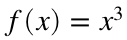. Here let's look at a concrete, but nontrivial function. Consider the functions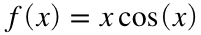on the interval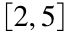. Suppose we are looking for the local extrema of this function; i.e., we look for the roots of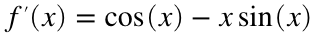.
Lets first plot the two functions to give us a graphical idea of what is happening.
x = [2:0.001:5];
f = x.*cos(x);
df = cos(x) - x.*sin(x);
plot(x, f, x, df, 'linewidth', 4);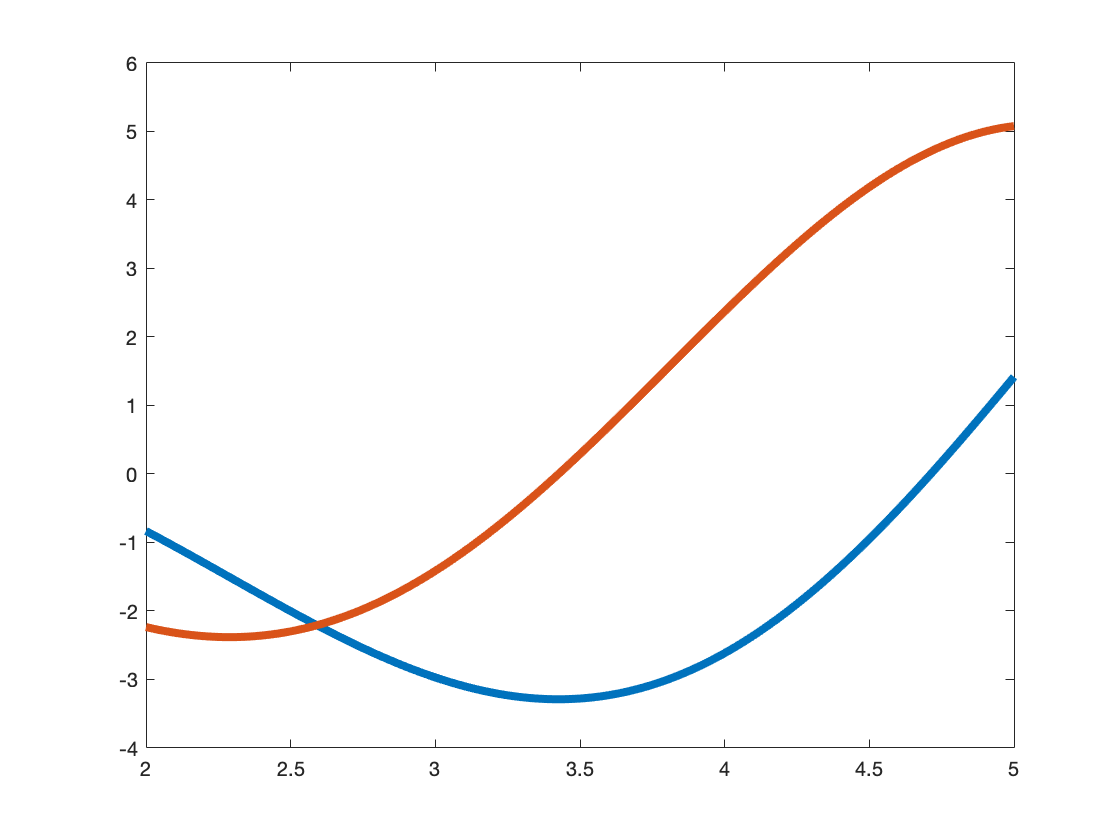### Section Search: Iterate and Pray

Notice that we have a discretization of x already in the plot above. And from that we have a discretization of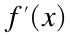. Now all we have to do is find the entry of x that corresponds tothat is closest to zero. In order to do that all we have to do is find the entry that gives us the minimum of the absolute value of.
x = [2:0.001:5];
f = x.*cos(x);
df = cos(x) - x.*sin(x);
[~, root_entry] = min(abs(df));
root = x(root_entry);
[root, df(root_entry)]
ans = 1×2
3.4260 0.0015
plot(x, f, x, df, 'linewidth', 4)
hold on
plot(root, 0, 'k.', root, f(root_entry), 'g.', 'Markersize', 40)
hold off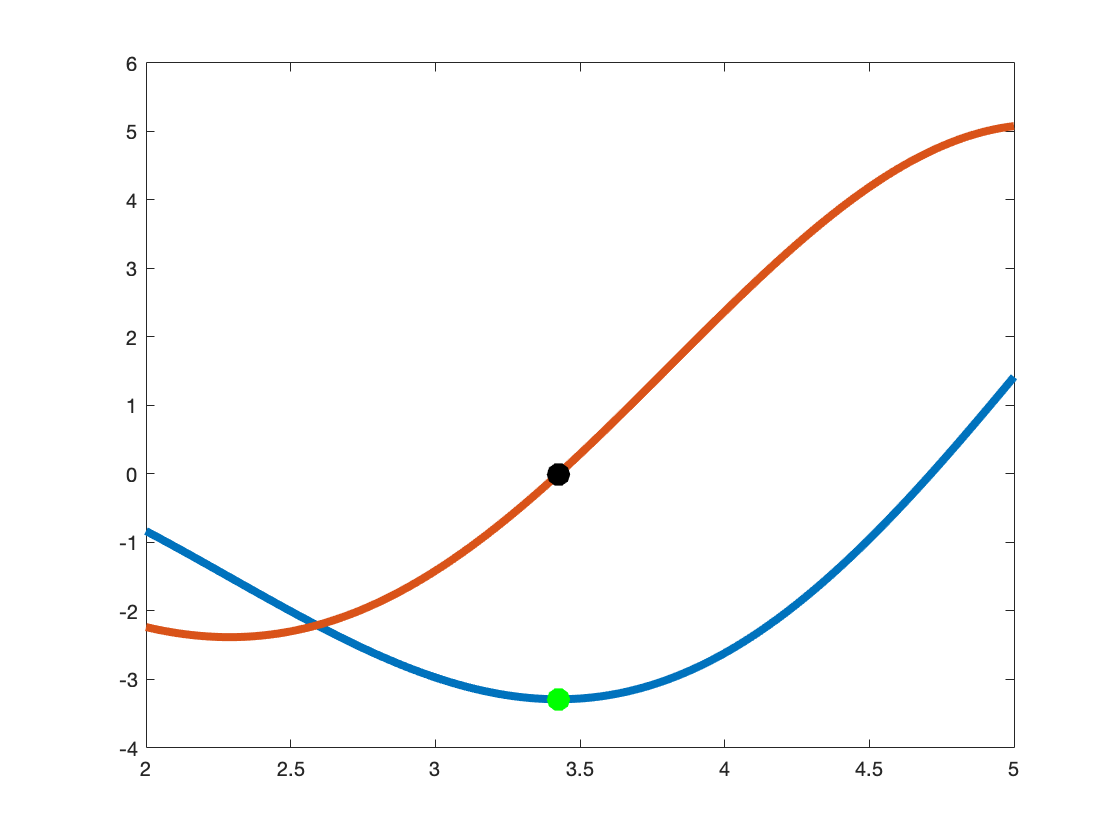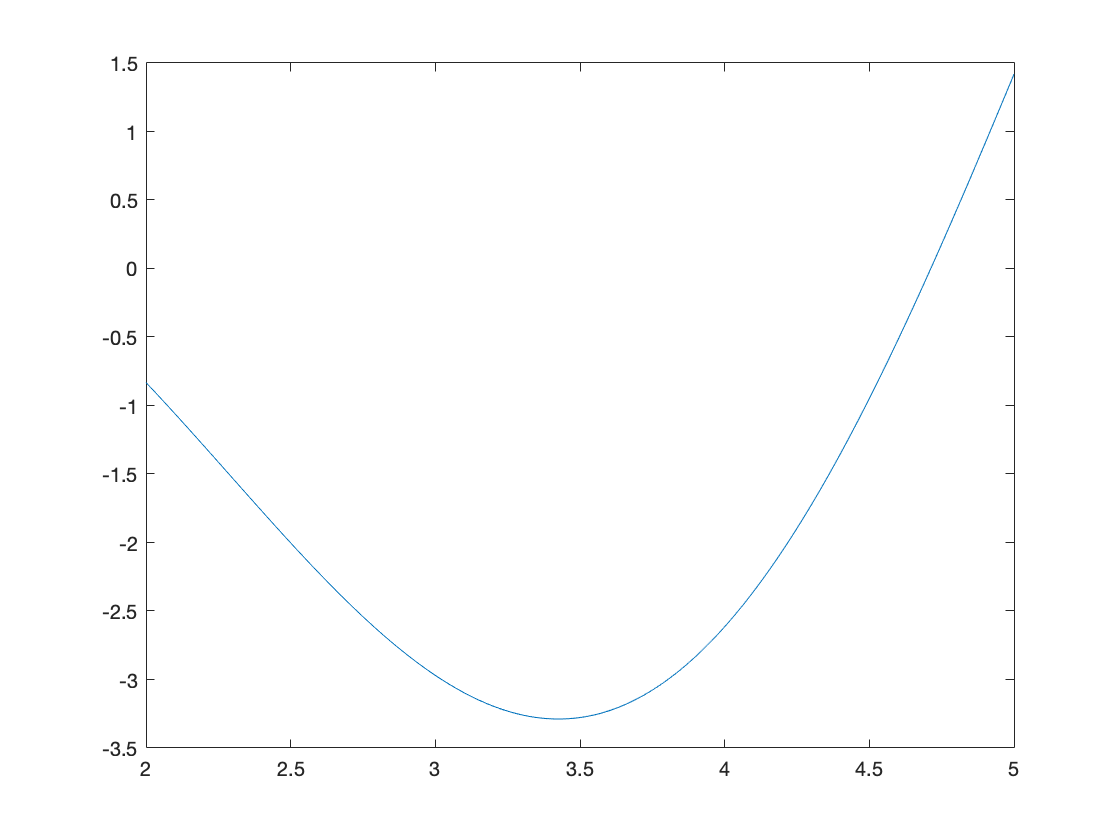Remeber, these are just approximations. That is not far off! But that is because we use a quite fine discretization. Let's try it with a much coarser discretization. This time it's quite far off the root, and since it's quite far of the root, can we even trust the local minima?
x = [2:0.5:5];
f = x.*cos(x);
df = cos(x) - x.*sin(x);
[~, root_entry] = min(abs(df));
root = x(root_entry);
[root, df(root_entry)]
ans = 1×2
3.5000 0.2913
plot(x, f, x, df, 'linewidth', 4)
hold on
plot(root, 0, 'k.', root, f(root_entry), 'g.', 'Markersize', 40)
hold off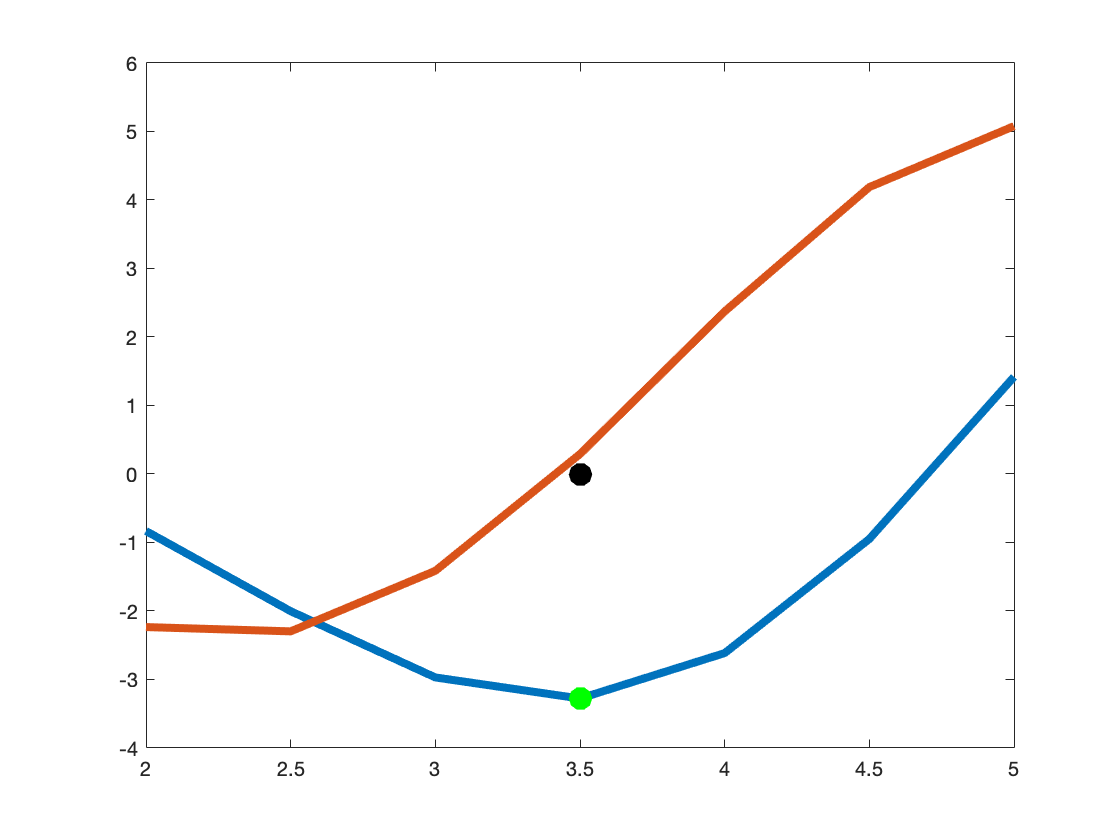Now what if we weren't restriced to a domain where the functiononly has one root? Say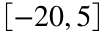.
x = [-20:0.001:5];
df = cos(x) - x.*sin(x);
plot(x, df, 'linewidth', 4);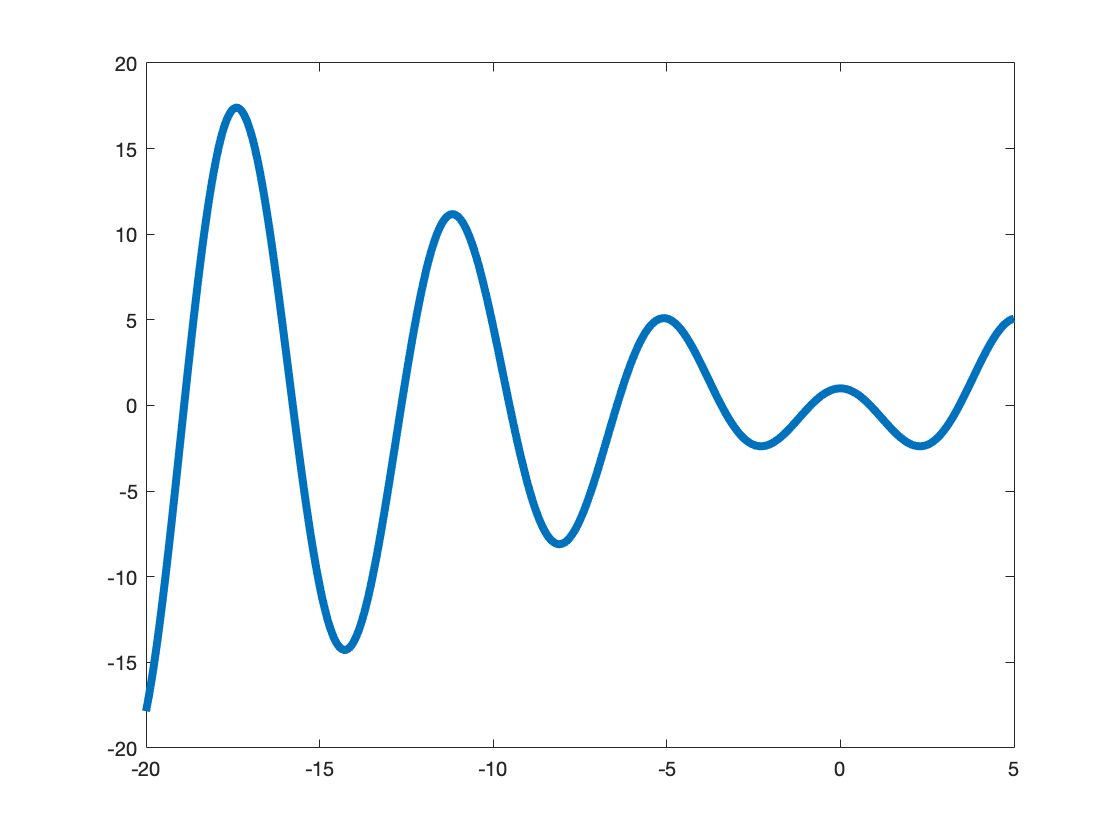Notice that this has quite a few roots. How do we find them all? This requires us to be a bit clever. Let's do a for loop that goes through the entirety of x, and pick out the points when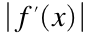is decreasing and then suddenly changes direction to increasing. Right after it changes direction we will pick the entry before it. This is like going over the edge, and then figure out the edge was just one step behind you.
x = [-20:0.001:5];
df = cos(x) - x.*sin(x);
for i = 1:length(x)-2
if abs(df(i+2)) > abs(df(i+1)) && abs(df(i)) > abs(df(i+1))
[x(i+1), df(i+1)]
end
end
ans = 1×2
-18.9020 0.0078
ans = 1×2
-15.7710 -0.0045
ans = 1×2
-12.6450 0.0037
ans = 1×2
-9.5290 -0.0032
ans = 1×2
-6.4370 0.0020
ans = 1×2
-3.4260 0.0015
ans = 1×2
-0.8600 0.0007
ans = 1×2
0.8600 0.0007
ans = 1×2
3.4260 0.0015

### Bisection Method

With bisection we must know that there is a root between two points a and b. If there are multiple roots, it will only find one.
For the interval, we know for sure that there is a root between 2 and 5. So we will bisect our interval each time until we hone in on a root.
a = 2;
b = 5;
df_a = cos(a) - a*sin(a);
df_b = cos(b) - b*sin(b);
xmid = (a+b)/2;
df_mid = cos(xmid) - xmid*sin(xmid);
for i = 1:10
if df_mid == 0
break
else if sign(df_mid) == sign(df_a)
a = xmid;
else
b = xmid;
end
end
xmid = (a+b)/2;
df_mid = cos(xmid) - xmid*sin(xmid);
df_a = cos(a) - a*sin(a);
df_b = cos(b) - b*sin(b);
end
format long
[xmid, cos(xmid) - xmid*sin(xmid)]
ans = 1×2
3.425292968750000 -0.001252652288744
a = 2;
b = 5;
df_a = cos(a) - a*sin(a);
df_b = cos(b) - b*sin(b);
xmid = (a+b)/2;
df_mid = cos(xmid) - xmid*sin(xmid);
for i = 1:100
if df_mid == 0
break
else if sign(df_mid) == sign(df_a)
a = xmid;
else
b = xmid;
end
end
xmid = (a+b)/2;
df_mid = cos(xmid) - xmid*sin(xmid);
df_a = cos(a) - a*sin(a);
df_b = cos(b) - b*sin(b);
end
format long
[xmid, cos(xmid) - xmid*sin(xmid)]
ans = 1×2
3.425618459481728 0.000000000000000

### Newton's method

With Newton's method we only need a nearby point to the root, however, we better be sure it's close to that root and not some other root. And even then it may not converge.
x = 5;
for i = 1:10
x = x - (cos(x) - x*sin(x))/(-2*sin(x) - x*cos(x)); %We do have to calculate the derivative here
end
[x, cos(x) - x*sin(x)]
ans = 1×2
-15.771284874815882 -0.000000000000007
x = 4;
for i = 1:10
x = x - (cos(x) - x*sin(x))/(-2*sin(x) - x*cos(x)); %We do have to calculate the derivative here
end
[x, cos(x) - x*sin(x)]
ans = 1×2
3.425618459481728 0.000000000000000

### Brief word on built in functions

Built in functions are useful, and it also allows you to not have to think too much about the problem at hand, but we should make sure not to be lulled into a sense of comfort. After all, these are just computer programs, not magic. I personally, for my work, only use built in functions if I know for sure it is optimized (e.g. on MATLAB most Linear Algebra functions are more optimized than something I could write, especially since I am not an expert in Linear Algebra). I am an expert in Differential Equations, and in my work I rarely ever use a built in differential equation solver because I often need it to do something specialized that I know for sure the creators of the solver did not optimize. Further, as we have seen with the backslash operator, we need to know how it works. If we don't we can very easily make irrepreparable damage to our codes (or worse, someone else's code that we are contributing to).
You will probably not have a need to use the following function, but it is there if on the off chance you need it. The following function looks for the local minima of our original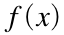between two points, similar to bisection. Here we use 2 and 5. It takes in an anonymous function and the two bounds 2 and 5.
fminbnd(@(x)(x * cos(x)), 2, 5)
ans =
3.425634147697436
The next function searches for local minima near a particular point, similar to Newton's method. Here we will use x = 5, y = 7. It takes in an anonymous function, and the nearby x and y values
f = @(v)((v(1) - 1)^2 + 3*(v(2) + 2)^2);
fminsearch(f, [5; 7])
ans = 2×1
1.000016192604872 -1.999979249216309
They work fine, but since we have to use anonymous functions, which are ridiculously slow for large operations, we are probably better off just writing our own code.